# Resistivity Laws of Resistance and Unit of Resistivity

## Resistivity or Coefficient of Resistance

Resistivity or Coefficient of Resistance is a property of substance, due to which the substance offers opposition to the flow of current through it. Resistivity or Coefficient of Resistance of any substance can easily be calculated from the formula derived from Laws of Resistance.

## Laws of Resistance

The resistance of any substance depends on the following factors,

1. Length of the substance.
2. Cross sectional area of the substance.
3. The nature of material of the substance.
4. Temperature of the substance.

There are mainly four (4) laws of resistance from which the resistivity or specific resistance of any substance can easily be determined.

#### First Law of Resistivity

The resistance of a substance is directly proportional to the length of the substance. electrical resistance R of a substance is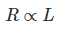Where L is the length of the substance.
If the length of a substance is increased, the path traveled by the electrons is also increased. If electrons travel long, they collide more and consequently the number of electrons passing through the substance becomes less; hence current through the substance is reduced. In other words, the resistance of the substance increases with increasing length of the substance. This relation is also linear.

#### Second Law of Resistivity

The resistance of a substance is inversely proportional to the cross-sectional area of the substance. Electrical resistance R of a substance is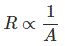Where A is the cross-sectional area of the substance.
The current through any substance depends on the numbers of electrons pass through a cross-section of substance per unit time. So, if the cross section of any substance is larger then more electrons can cross the cross section. Passing of more electrons through a cross-section per unit time causes more current through the substance. For fixed voltage, more current means less electrical resistance and this relation is linear.

## Resistivity

Combining these two laws we get,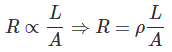Where, ρ (rho) is the proportionality constant and known as resistivity or specific resistance of the material of the conductor or substance. Now if we put, L = 1 and A = 1 in the equation, we get, R = ρ. That means resistance of a material of unit length having unit cross – sectional area is equal to its resistivity or specific resistance. Resistivity of a material can alternatively be defined as the electrical resistance between opposite faces of a cube of unit volume of that material.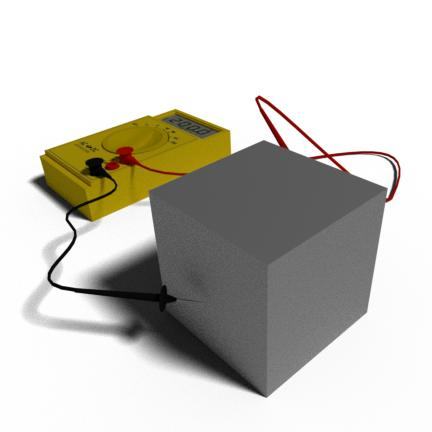#### Third Law of Resistivity

The resistance of a substance is directly proportional to the resistivity of the materials by which the substance is made. The resistivity of all materials is not the same. It depends on the number of free electrons, and size of the atoms of the materials, types of bonding in the materials and many other factors of the material structures. If the resistivity of a material is high, the resistance offered by the substance made by this material is high and vice versa. This relation is also linear.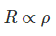#### Fourth Law of Resistivity

The temperature of the substance also affects the resistance offered by the substance. This is because, the heat energy causes more inter-atomic vibration in the metal, and hence electrons get more obstruction during drifting from lower potential end to higher potential end. Hence, in metallic substance, resistance increases with increasing temperature. If the substance in nonmetallic, with increasing temperature, the more covalent bonds are broken, these cause more free electrons in the material. Hence, resistance is decreased with increase in temperature.
That is why mentioning resistance of any substance without mentioning its temperature is meaningless.

### Unit of Resistivity

The unit of resistivity can be easily determined form its equation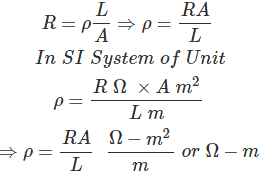The unit of resistivity is Ω – m in MKS system and Ω – cm in CGS system and 1 Ω – m = 100 Ω – cm.

### List of Resistivity of Different Commonly Used Materials

 Materials Resistivity in μ Ω – cm at 20oC Aluminium 2.82 Brass 6 to 8 Carbon 3k to 7k Constantan 49 Copper 1.72 Gold 2.44 Iron 12.0 Lead 22.0 Manganin 42 to 74 Mercury 96 Nickel 7.8 Silver 1.6 Tungsten 5.51 Zinc 6.3

Want To Learn Faster? 🎓
Get electrical articles delivered to your inbox every week.
No credit card required—it’s 100% free.